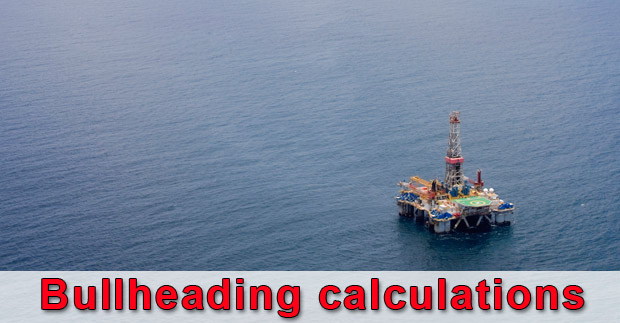Components of Pumping Pressure

For the bullheading operation, pumping pressure on surface is equal to summation of all frictional pressure and formation pressure minus hydrostatic pressure (Figure 1). The equation below shows this relationship in a mathematical term.

Pump Pressure = Friction Pressure of Surface Lines + Friction Pressure of Tubing + Friction Pressure Across Perforations + Formation Pressure – Hydrostatic Pressure of Tubing

The pump pressure concept will be utilized for the bull heading calculation.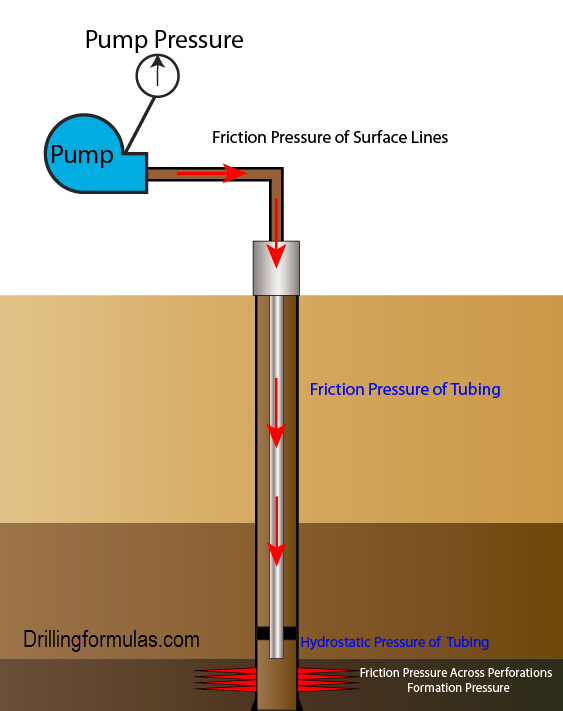Figure 1 – Pump Pressure Components

The well information is give below;

Production casing was set at 12,000’MD/12,000’TVD.

Bottom of perforation is at 11,500’MD/11,500’TVD.

Bottom of perforation is at 11,000’MD/11,000’TVD.

End of production tubing is at 11,500’MD/11,500’TVD.

Production packer is at 10,300’MD/10,300’TVD.

Formation fracture gradient is 0.645 psi/ft

Formation pressure gradient is 0.445 psi/ft

Shut in tubing pressure = 2,800 psi

Production casing: 7” OD, 29 ppf, L-80, capacity factor = 0.0371 bbl/ft

Production tubing: 3.5” OD, 9.2ppf, L-80, capacity factor = 0.0087 bbl/ft

Pump output (bbl/stk) = 0.1 bbl/stk

Figure 2 describes the wellbore diagram based on the given information.Figure 2 – Diagram of the well

Calculations

For the bull heading calculation, reference points for calculation formation pressure, fracture pressure, kill weight mud are based on top of perforation because it gives the most conservative fracture pressure value.

Formation Pressure (psi) = Pressure Gradient (psi/ft) x Top of Perforation TVD (ft)

Formation Pressure (psi) = 0.445 x 11,000 = 4,895 psi

Fracture Pressure (psi) = Fracture Gradient (psi/ft) x Top of Perforation TVD (ft)

Fracture Pressure (psi) = 0.645 x 11,000 = 7,095 psi

Initial Hydrostatic Pressure (psi) = Formation Pressure (psi) – Shut In Tubing Head Pressure (psi)

Initial Hydrostatic Pressure (psi) = 4,895 – 2,800 = 2,095 psi

Initial Average Fluid Density (ppg) = Initial Hydrostatic Pressure (psi) ÷ (0.052 x Top of Perforation TVD (ft))

Initial Average Fluid Density (ppg) = 2,095 ÷ (0.052 x 11,000) = 3.66 ppg

Kill Weight Mud (ppg) = Initial Average Fluid Density + (Shut In Tubing Pressure (psi) ÷ 0.052 ÷ Top of Perforation TVD (ft))

Kill Weight Mud (ppg) = 3.66 + (2800÷ 0.052 ÷11,000) = 8.6 ppg

Maximum Initial Surface Pressure (psi) = Formation Fracture Pressure (psi) –Initial Hydrostatic Pressure (psi)

Maximum Initial Surface Pressure (psi) = 7,095 – 2,095 = 5,000 psi

The below calculation relates to pressure while bullheading.

Maximum End of Tubing Pressure (psi) = Fracture Pressure (psi) – (Kill Weight Mud (ppg) x 0.052 x End of Tubing TVD (ft)) – Initial Average Fluid Density (ppg) x 0.052 x (Top of Perforation TVD (ft) – End of Tubing TVD (ft))

Maximum End of Tubing Pressure (psi) = 7,095 – (8.6 x 0.052 x 10,500) – (3.66 x 0.052 x (11,000 – 10,500)) = 2,304 psi

Maximum pressure when Kill Mud Weight reaches perforation

@ Top of Perforation (11,000 ft TVD)

Maximum Final Pressure (psi) = Formation Fracture Pressure @ top of perforation (psi) – (Kill Weight Mud (ppg) x 0.052 x Top of Perforation TVD (ft))

Maximum Final Pressure (psi) = 0.645 x 11,000 – (8.6 x 0.052 x 11,000)

Maximum Final Pressure (psi) = 2,176 psi

@ Bottom of Perforation (11,500 ft TVD)

Maximum Final Pressure (psi) = Formation Fracture Pressure @ bottom of perforation (psi) – (Kill Weight Mud (ppg) x 0.052 x Bottom of Perforation TVD (ft))

Maximum Final Pressure (psi) = 0.645 x 11,500 – (8.6 x 0.052 x 11,500)

Maximum Final Pressure (psi) = 2,275 psi

The most conservative figure for the maximum final pressure is 2,176 psi.

As you can see, the figure reference to the top of perforation gives the most conservative figure. This is the reason why top of perforation is selected for the calculation.

Volume Pumped in Tubing (bbl) = Tubing Capacity Factor (bbl/ft) x Length of Tubing (ft)

Volume Pumped in Tubing (bbl) = 0.0087 x 10,500 = 91.4 bbl

Stroke Pumped in Tubing (stk) = Volume Pumped in Tubing (bbl) ÷ Pump Output (bbl/strk)

Volume Pumped in Tubing (stk) = 91.4 ÷ 0.1 = 914 strokes

Volume Pumped From End of Tubing to Top of Perforation (bbl) = Casing Capacity Factor (bbl/ft) x (Top of Perforation TVD (ft) –End of Tubing TVD (ft))

Volume Pumped in Tubing (bbl) = 0.0317 x (11,000 – 10,500) = 18.6 bbl

Stroke Pumped From End of Tubing to Top of Perforation (stk) = Volume Pumped in Casing (bbl) ÷ Pump Output (bbl/strk)

Volume Pumped in Tubing (stk) = 18.6 ÷ 0.1 = 186 strokes

Volume Pumped From Top of Perforation to End of Perforation (bbl) = Casing Capacity Factor (bbl/ft) x (End of Perforation TVD (ft) – Top of Perforation TVD (ft))

Volume Pumped in Tubing (bbl) = 0.0317 x (11,500 – 11,000) = 18.6 bbl

Stroke Pumped From Top of Perforation to End of Perforation (bbl)) = Volume Pumped in Casing (bbl) ÷ Pump Output (bbl/strk)

Volume Pumped in Tubing (stk) = 18.6 ÷ 0.1 = 186 strokes

Total Volume Pumped Summary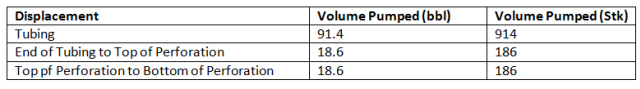In order to push all formation fluid back to formation, it is required that the pumping volume must be at least volume from surface to end of perforation.

This is the same concept as pressure schedule in wait and weight well control method.

Pressure Decreasing in Tubing (psi/required stks) = (Maximum Initial Surface Pressure (psi) – Maximum End of Tubing Pressure (psi)) X Required strokes (stks) ÷ Tubing volume (stk)

For this calculation, 100-strokes is selected.

Pressure Decreasing in Tubing (psi/required stks) = (5,000 – 2,304) x 100 ÷ 914 = 295 psi / 100 stks

Pressure Decreasing in Casing (psi/required stks) = (Maximum Initial End of Tubing Pressure (psi) – Maximum Final Pressure (psi)) X Required strokes (stks) ÷  Volume from End of Tubing to Top of Perforation (stk)

Pressure Decreasing in Casing (psi/required stks) = (2,304 – 2,176) X 100 ÷ 186 = 96psi / 100 stks

Draw the bullhead chart based on this data

The red line is the maximum pressure. If pressure exceeds the red line, a formation will be broken down (fracture zone). The blue line represents a shut in condition. Pressure below the blue line means that the well is in an underbalanced condition (flow zone). The area between the red line and blue line is the safe zone for bullheading operation (Figure 3).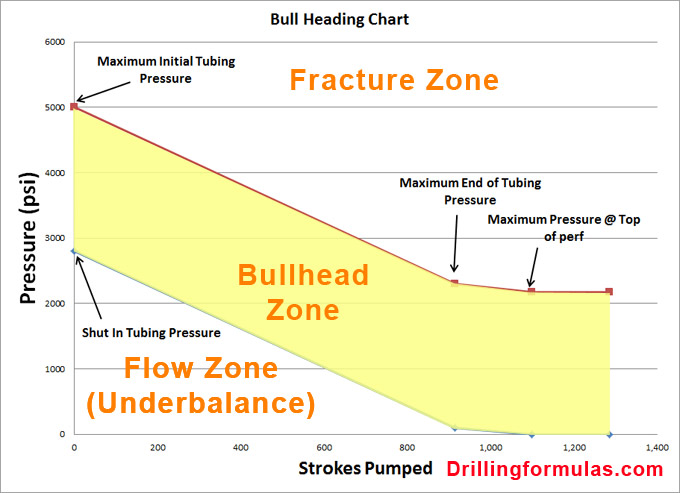For safe operation, pumping pressure must be within the bullheading zone (Figure 4).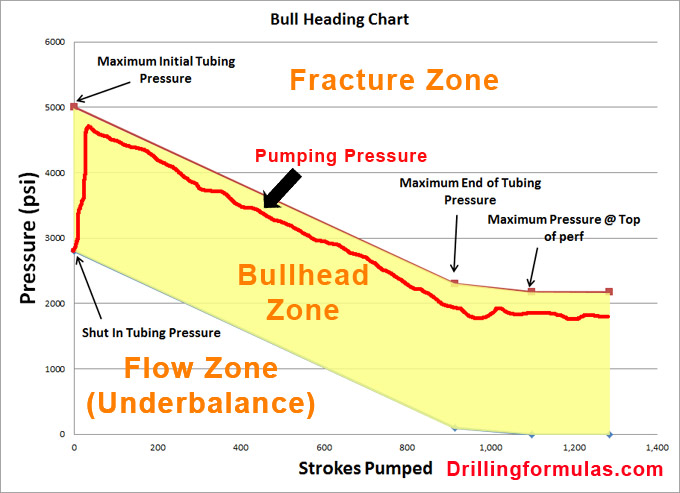The formations may be fractured if pumping pressure exceeds the fracture line (Figure 5).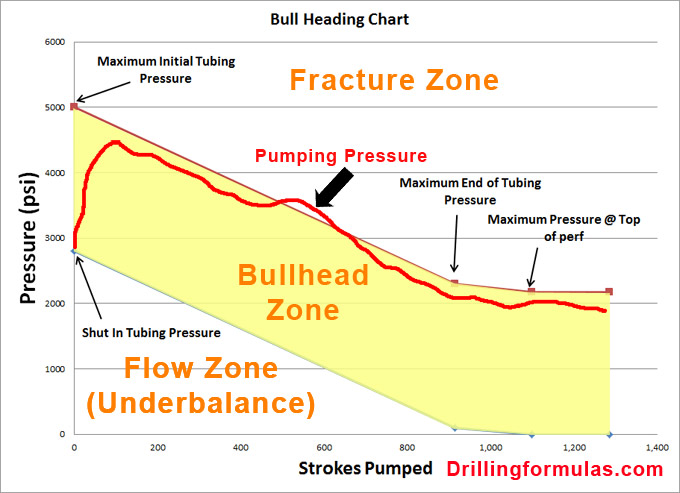Figure 5 -Bullheading Operation Exceeding Fracture Pressure

Note: this chart is constructed without accounting for friction pressure. It is the most conservative pressure to prevent fracturing formation.

References

Cormack, D. (2007). An introduction to well control calculations for drilling operations. 1st ed. Texas: Springer.

Crumpton, H. (2010). Well Control for Completions and Interventions. 1st ed. Texas: Gulf Publishing.

Grace, R. (2003). Blowout and well control handbook [recurso electrónico]. 1st ed. Paises Bajos: Gulf Professional Pub.

Grace, R. and Cudd, B. (1994). Advanced blowout & well control. 1st ed. Houston: Gulf Publishing Company.

Share the joy
Tagged , . Bookmark the permalink.Working in the oil field and loving to share knowledge.

### 5 Responses to Bullheading Calculation Example

1.Parveen Kumar Sharma says:

Excelent formulas and best sight for the oil field employees To gain knowledge.
Thank’s & Regd

2.mujiono says:

3.Luis Alberto Barrios Villa says:

Very good help for all people whon still working on oil field!!

4.Luis Alberto Barrios Villa says:

Very good help for all people whom still working on oil field!!

5.Mangalsinghrana01@gmail.com says:

Thanks for helping bull heading formula – MS Rana

This site uses Akismet to reduce spam. Learn how your comment data is processed.